# Portfolio risk: Variance and covariance

4.2You now know that to measure risk, we need to calculate the variance (and by extension, the standard deviation). So, the question we now have to answer is this - How do you measure portfolio variance? There are many layers to this. But as always, we’ll begin with something simple.

Let’s take a two-asset portfolio to get started.

### Measuring the variance of a two-asset portfolio

You have a two-asset portfolio with two stocks: stock A and stock B. For this portfolio, here’s the formula you can use to calculate the variance.

 Portfolio variance = wA2σA2 + wB2σB2 + 2wAwBCovA,B

This is what the formula means:

• wA = The weight of stock A in the portfolio
• σA  = The standard deviation of stock A
• wB = The weight of stock Bin the portfolio
• σB  = The standard deviation of stock B
• CovA,B = The covariance of stock A and stock B

The covariance has its own formula, which goes as follows:

 Covariance = p(A,B)σAσB

Here, p(A,B) represents the correlation coefficient of the two stocks.

Okay, you now know the variance and covariance formula. But let’s back up a bit. There are some terms here that we need to decode first. What is covariance? And what is the correlation coefficient?

### Covariance:

Covariance is a measure of how two variables are related to one another. It tells you how two random variables change together. Covariance can be a positive number or a negative number. A positive covariance means that the two variables move in the same direction. And a negative covariance means that they move in opposite directions.

### Correlation coefficient:

Remember reading up about this in the module on pair trading? The correlation coefficient is a measure of the correlation between two variables. The correlation shows the strength of the relationship between the variables.

The correlation coefficient ranges from -1 to +1. The symbol tells you the direction of movement. A positive symbol means that the two variables tend to move in the same direction, while a negative symbol means they typically move in opposite directions. The number following the symbol tells you the strength of the correlation. So, for example, a correlation coefficient of 0.95 is stronger than a correlation coefficient of 0.38.

So, coming back to measuring the variance of a two-asset portfolio, let’s take up this set of hypothetical data.

• You have two assets in the portfolio: stock A and stock B.
• You invest 40% of your capital in stock A and 60% of it in stock B.
• The standard deviation of stock A is 2%.
• The standard deviation of stock B is 3%.
• The correlation coefficient of the two stocks is 0.90.

In this case, using the formula for portfolio variance, this is what we get.

Portfolio variance:

= wA2σA2 + wB2σB2 + 2wAwBCovA,B

= (40%2 x 2%2) + (60%2 x 3%2) + (2 x 40% x 60% x 2% x 3% x 0.90)

= 0.064%

From this, you can arrive at the standard deviation of the portfolio, which is the square root of the variance.

So, the standard deviation of the portfolio = Square root of 0.064%

This comes in at 2.52%.

### Measuring the variance of a multi-asset portfolio

Making the calculations for two assets is easy. But what about portfolios that have multiple assets? Calculating the covariance, the correlation coefficient and the variance with multiple assets using the formula is exhausting. Here’s where the excel tool comes in handy. To calculate the variance and eventually the risk of a multi-asset portfolio, we need to construct a variance-covariance matrix and then, a correlation matrix.

All in good time. First, let’s see how you can calculate the covariance for 2 stocks using excel. There is, of course, a direct formula. But you can also do it step-wise. We’ll take a look at both the approaches using a set of hypothetical data.

Step 1: Collect the variable data points for the two stocks.

Here, we’ll take the prices of two stocks - stock A and stock B. Remember that this is just a set of hypothetical data. You can download the actual prices of the stocks from the NSE’s or the BSE’s website for the period you wish to consider. Ideally, it’s a good idea to take data worth at least 6 months. 1 year’s worth of data would be more ideal.

For this example, we’ve just taken 10 data points to make calculations easier. Check out the screenshot here, showing the prices of stock A and stock B over 1 10-day period.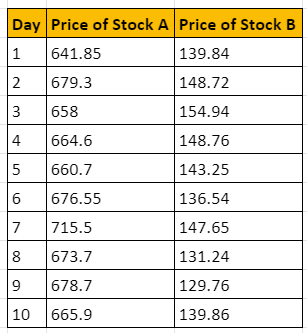Step 2: Calculate the daily returns for the two assets

Recall how we did this for pair trading? The daily return is calculated as the difference in return between two days, divided by the base return. Check it out here.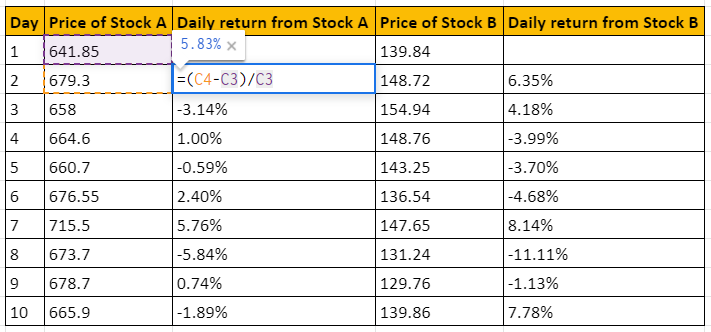Step 3: Calculate the average daily return for the two stocks

The average of the daily returns for each stock is essentially the mean. This is a simple enough calculation. As you can see from the image below, the average returns of stock A comes in at 0.48%, and the returns from stock B comes in at 0.20%.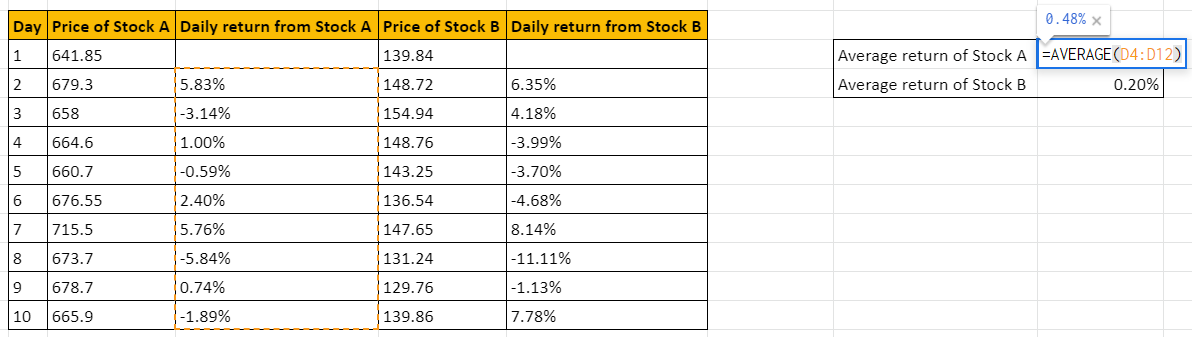Step 4: Calculate the deviation of each variable from the mean

This is simply the difference between each day’s return, and the average return.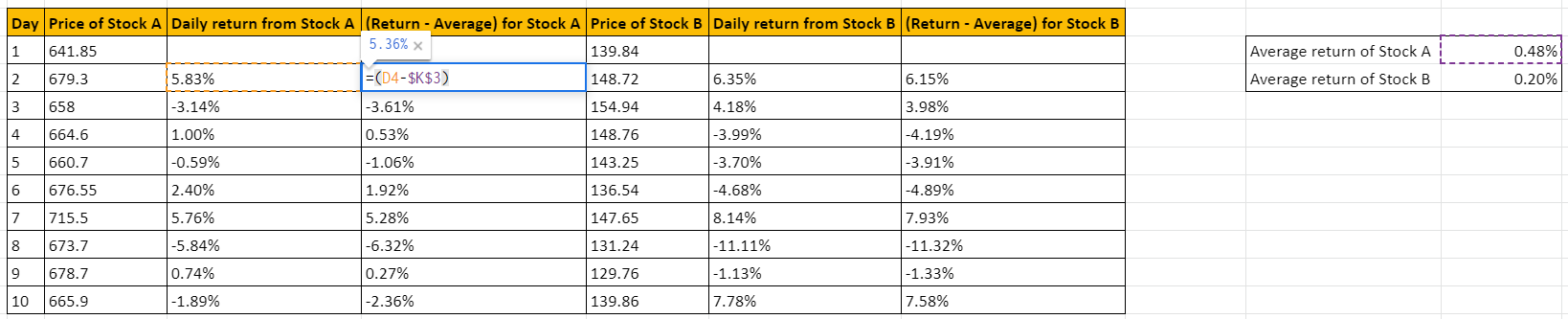Step 5: Calculate the covariance

There is a formula for the covariance in excel. So, we’ll just use that to get the value of this measure. As you can see, the covariance comes up to 0.00118063.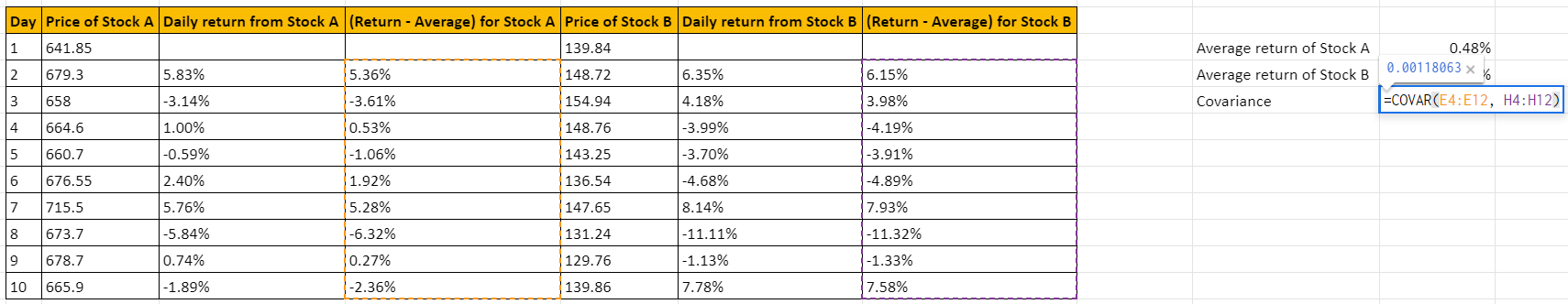The covariance is positive, so it indicates that the prices of these two stocks are moving in the same direction.

#### Wrapping up

Like this, we can also use the tools in excel to calculate the risk of a portfolio. But to do that, we need to get a little bit into mathematics and understand the basics of matrices. Ready to do that? Check out the next chapter then, where we introduce you to matrix mathematics. If you’ve already learned this in school, this will be a good refresher.

#### A quick recap

• The portfolio variance of a two-asset portfolio is calculated using the formula: w12σ12 + w22σ22 + 2w1w2Cov1,2
• Covariance is a measure of how two variables are related to one another. It tells you how two random variables change together.
• Covariance can be a positive number or a negative number.
• A positive covariance means that the two variables move in the same direction.
• And a negative covariance means that they move in opposite directions.
• The correlation coefficient is a measure of the correlation between two variables.
• The correlation shows the strength of the relationship between the variables.
• The correlation coefficient ranges from -1 to +1.
• The symbol tells you the direction of movement.
• A positive symbol means that the two variables tend to move in the same direction, while a negative symbol means they typically move in opposite directions.
• The number following the symbol tells you the strength of the correlation.
• To calculate the variance and eventually the risk of a multi-asset portfolio, we need to construct a variance-covariance matrix and then, a correlation matrix.